# SSAT Elementary Level Math : How to add

## Example Questions

← Previous 1 3 4 5 6 7 8 9 161 162

### Example Question #1 : How To Add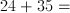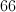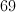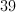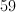Explanation:

Line the numbers up, one above the other.  Add 4 and 5 to get 9.  Add 2 and 3 to get 5.  The answer is 59.

### Example Question #2 : How To Add

Round 2342 to the nearest hundred.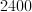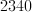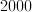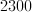Explanation:

First, identify which number is in the hundreds place.  The hundreds place is 3 places to the left of the decimal.  In this case, 3 is in the hundreds place.

If the numbers to the right of the 3 are 50 or more, then we will round it up to 4.  If they are less than 50, we will leave the 3 the same.  Here, we have 42, so the 3 will remain the same.  The two numbers to the right become zeroes.

We round 342 down to 300.  The 2 in the thousands place remains the same.

### Example Question #1 : Fluently Add, Subtract, Multiply, And Divide Multi Digit Decimals: Ccss.Math.Content.6.Ns.B.3

Fill in the blank to make a true statement:

_______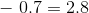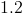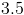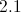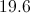Explanation:

To find out what number to subtract 0.7 from to get 2.8, just do the opposite - add 0.7 to 2.8.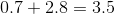### Example Question #3 : How To Add

Put the following numbers in order from least to greatest: 7, 3, 6, 1

7, 1, 3, 6

7, 3, 6, 1

1, 3, 7, 6

7, 6, 3, 1

1, 3, 6, 7

1, 3, 6, 7

Explanation:

The least number is 1, followed by 3, then 6 and finally, 7.

### Example Question #1 : How To Add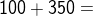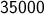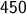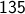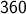Explanation: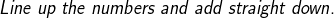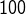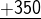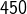### Example Question #5 : How To Add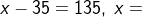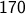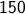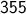Explanation: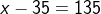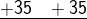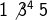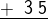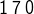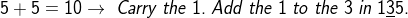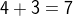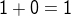### Example Question #6 : How To Add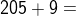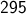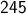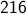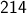Explanation: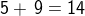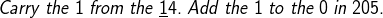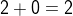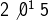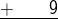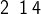### Example Question #7 : How To Add

Find the sum.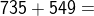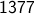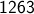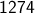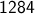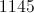Explanation:

Line up the numbers vertically. Add the ones place first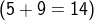. Keep the 4, carry the 1. Then add the 3 digits in the tens place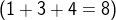. Keep the 8. Then add the hundreds place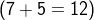. The sum is 1284

### Example Question #8 : How To Add

If Laura has three quarters, two dimes, and one nickel, how much money does she have?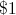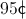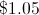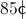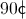Explanation:

One quarter is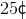, one dime is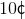, and one nickel is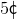.

Three quarters is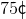, 2 dimes is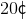, and one nickel is. If you add these three values you get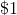.

### Example Question #1 : Adding Multi Digit Decimals

Find the sum of 2.4, 55, and 6.21.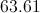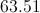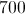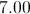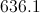Explanation:

To find the sum, you need to add. Line up all numbers vertically. Since one number has a decimal to the tenths place, one has a decimal to the hundredths place, and one has no decimal, be extra careful to line up the digits correctly! To help you, add a zero to the end of 2.4, and add two zeroes to the end of 55 so that all the digits line up correctly.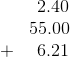Now, add down each column, starting on the right side and moving to the left.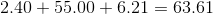The sum is 63.61.

← Previous 1 3 4 5 6 7 8 9 161 162